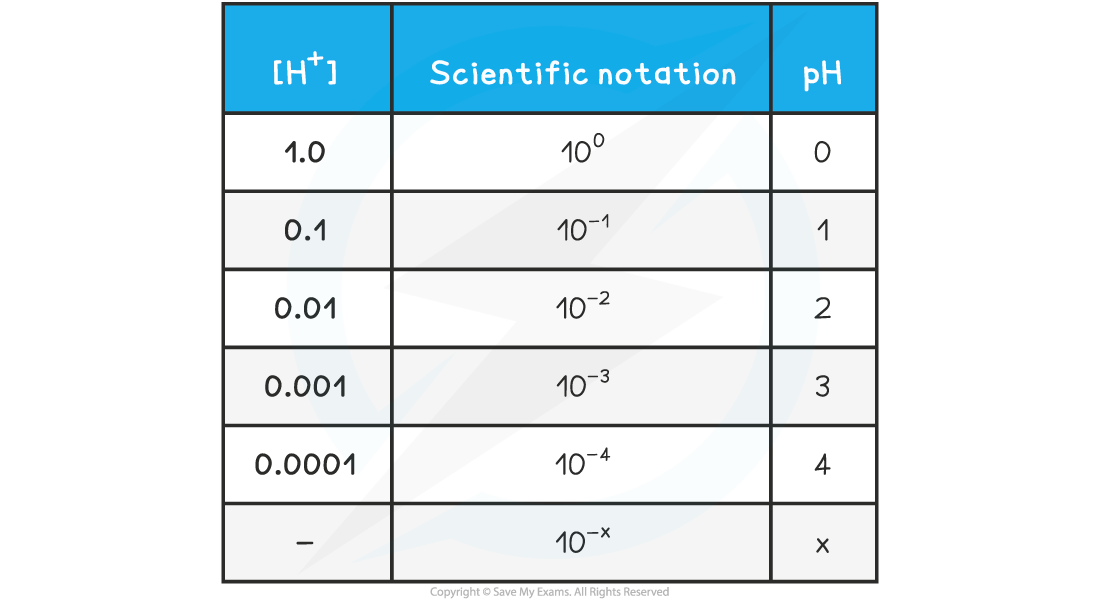# Edexcel A Level Chemistry:复习笔记5.2.2 pH

### Defining pH

• The acidity of an aqueous solution depends on the number of H+ ions in solution
• The pH is defined as:

pH = -log[H+]

• where [H+] is the concentration of hydrogen ions in mol dm–3
• Similarly, the concentration of H+ of a solution can be calculated if the pH is known by rearranging the above equation to:

[H+] = 10-pH

• The pH scale is a logarithmic scale with base 10
• This means that each value is 10 times the value below it. For example, pH 5 is 10 times more acidic than pH 6.
• pH values are usually given to 2 decimal places
• The relationship between concentration is easily seen on the following table

pH & [H+] Table### pH & Hydrogen Ion Concentration

#### Worked Example

pH and H+ calculations

Question 1: Find the pH when the hydrogen concentration is 1.60 x 10-4 mol dm-3

Question 2: Find the hydrogen concentration when the pH is 3.10

The pH of the solution is:

pH  = -log[H+]

= -log 1.6 x 10-4

= 3.80

The hydrogen concentration can be calculated by rearranging the equation for pH

pH   = -log[H+]

[H+] = 10-pH

= 10-3.10

= 7.94 x 10-4 mol dm-3

#### Worked Example

Powers of 10

10.0 cm3 of an aqueous solution of an acid of pH = 1.0 is mixed with 990.0 cm3 of distilled water. What is the pH of the final solution?

A.  1

B.  2

C.  3

D.  10